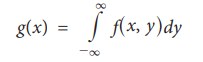Home | | Statistics 11th std | Joint and marginal probability mass functions

# Joint and marginal probability mass functions

In real life situations we may be observed two or more random variables on the individuals simultaneously.

Joint and marginal probability mass functions

In real life situations we may be observed two or more random variables on the individuals simultaneously. For instance, blood pressure and cholesterol for each individual are measured simultaneously. In such cases we require the concept of bi-variate random variable represented by ( X, Y ), where X and Y are univariate random variables.

## Definition (Joint p.m.f)

Let (X, Y) be a discrete bivariate random variable. Then p (x, y) is called the joint probability mass function of (X, Y) if the following conditions are satisfied.## Definition (Marginal Probability Mass Function)

Given a joint probability mass function p (x, y), then p (x) = Σy p(x,y) is called marginal probability mass function of X. Similarly p (y) = Σx p(x,y) is called the marginal probability mass function of Y.

### Example 9.14

There are 10 tickets in a bag which are numbered 1, 2, 3, ...10. Two tickets are drawn at random one after the other with replacement.

Here, random variable X denotes the number on the first ticket and random variable Y denotes the number on the second ticket.

## Joint and marginal probability density functions

As we defined in section 9.5.1 the joint probability mass function, we define the joint probability density function.

### Definition:

Let (X, Y) be a bivariate continuous random variables. The function f (x, y) is called a bivariate probability density if the following conditions are satisfied.The marginal probability density function of X is given byand the marginal probability density function of Y is given by### Example 9.15

Prove that the bivariate function given by f(x, y) =### Proof:

If f is a probability density functionTherefore, f (x, y) is a probability density function.

### Example 9.16

Joint p.d.f. of X,Y is f(x, y) =then find the marginal density function of X and Y.

### Solution:### Example 9.17

Joint p.d.f. of X,Y is f(x, y) =Find the marginal density function of X and Y

Solution:Study Material, Lecturing Notes, Assignment, Reference, Wiki description explanation, brief detail
11th Statistics : Chapter 9 : Random Variables and Mathematical Expectation : Joint and marginal probability mass functions |